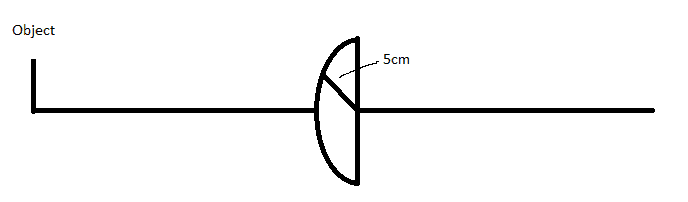# Ray transfer matrix - Plano convex lens

## Homework Statement

I have to find the ray transfer matrix for a Plano convex lens, with a 5 cm radius and refraction index n=1.8.
I'm supposed to use the paraxial approximation.
Attached a homemade picture of the lens.## Homework Equations

Refraction at a curved surface:
\begin{pmatrix}
1 & 0\\
\frac{n_1-n_2}{Rn_2} & \frac{n_1}{n_2}\\
\end{pmatrix}
Propagation matrix for the inside:
\begin{pmatrix}
1 & d\\
0 & 1\\
\end{pmatrix}
Refraction at a flat surface:
\begin{pmatrix}
1 & 0\\
0 & \frac{n_1}{n_2}\\
\end{pmatrix}

## The Attempt at a Solution

I would multiply the three matrices like this: $\begin{pmatrix} 1 & 0\\ 0 & \frac{n_1}{n_2}\\ \end{pmatrix} \begin{pmatrix} 1 & d\\ 0 & 1\\ \end{pmatrix} \begin{pmatrix} 1 & 0\\ \frac{n_1-n_2}{Rn_2} & \frac{n_1}{n_2}\\ \end{pmatrix}$

What is d? Is it 5 cm because it's a half sphere and all rays travel 5 cm?

Kind regards Alex :)

BvU
Homework Helper
∞Looks correct to me. "center thickness of lens" is d allright and R2 = ∞. You might want to check this thread.

@TSny appears to be the expert here.

Great thanks for the help but the result depends on the direction from which the ray comes. If it hits the flat surface I get the same result as the link you sent me but the other way, I get some other result: $\frac{n_2R}{n_2-n_1}$ with n_1=1. What result is correct?

Last edited:
TSny
Homework Helper
Gold Member
I would multiply the three matrices like this: $\begin{pmatrix} 1 & 0\\ 0 & \frac{n_1}{n_2}\\ \end{pmatrix} \begin{pmatrix} 1 & d\\ 0 & 1\\ \end{pmatrix} \begin{pmatrix} 1 & 0\\ \frac{n_1-n_2}{Rn_2} & \frac{n_1}{n_2}\\ \end{pmatrix}$

Something to think about: Do n1 and n2 in the first matrix have the same values as n1 and n2 in the last matrix? In other words, what is the interpretation of n1 and n2 at a refracting surface?

What is d? Is it 5 cm because it's a half sphere and all rays travel 5 cm?

Yes, for paraxial rays, d can be approximated by the thickness of the lens.

@TSny appears to be the expert here.

A very kind remark. But, sadly, I know very little optics. However, I do have an old copy of Hecht and Zajac :)

Thanks for the replies.
Yea TSny that was my first mistake :) Corrected it:

$\begin{pmatrix} 1 & 0\\ 0 & \frac{n_1}{n_2}\\ \end{pmatrix} \begin{pmatrix} 1 & d\\ 0 & 1\\ \end{pmatrix} \begin{pmatrix} 1 & 0\\ \frac{n_2-n_1}{Rn_1} & \frac{n_2}{n_1}\\ \end{pmatrix}$
It is reversed in the last matrix because the ray goes from lens to air.

Then I get this:
$\begin{pmatrix} 1+\frac{d(n_2-n_1)}{Rn_1} & \frac{dn_2}{n_1}\\ \frac{n_2-n_1}{Rn_2} & 1\\ \end{pmatrix} then \frac{n_2-n_1}{Rn_2}=\frac{1}{f}$

If I do it the otherway around I get the other result:

$\begin{pmatrix} 1 & \frac{dn_1}{n_2}\\ \frac{n_2-n_1}{Rn_1} & \frac{d(n_2-n_1)}{Rn_2}+1\\ \end{pmatrix} then \frac{1}{f}=\frac{n_2-n_1}{Rn_1}$
Which result is correct when you consider the object is on the left side, facing the curved surface. (First matrix)?

TSny
Homework Helper
Gold Member
Refraction at a curved surface:
\begin{pmatrix}
1 & 0\\
\frac{n_1-n_2}{Rn_2} & \frac{n_1}{n_2}\\
\end{pmatrix}
In order to get the n1 and n2 in the right place, I find it useful to write the matrix as

\begin{pmatrix}
1 & 0\\
\frac{n_i-n_t}{Rn_t} & \frac{n_i}{n_t}\\
\end{pmatrix}
where ni is the index of refraction on the side of the surface where the light is incident on the surface and nt is the index on the other side of the surface (the transmitted side).

Thanks for the replies.
Yea TSny that was my first mistake :) Corrected it:

$\begin{pmatrix} 1 & 0\\ 0 & \frac{n_1}{n_2}\\ \end{pmatrix} \begin{pmatrix} 1 & d\\ 0 & 1\\ \end{pmatrix} \begin{pmatrix} 1 & 0\\ \frac{n_2-n_1}{Rn_1} & \frac{n_2}{n_1}\\ \end{pmatrix}$

It is reversed in the last matrix because the ray goes from lens to air.

The last matrix is the refraction matrix for the first surface, where the light goes from air into the lens. The last matrix will act first when operating on a ray. You have to get used to reading matrix products from right to left when they operate on a ray.

The overall matrix for the case of light hitting the flat surface first will not be the same as the case of light hitting the curved surface first, but you should get the same focal length for both cases.

Let's check our matrices again, I'm not getting the same result as you.

Thanks for sticking with me :) I did the calculation again:
For a ray incident on the curved surface:
$\begin{pmatrix} 1 & 0\\ 0 & \frac{n_2}{n_1}\\ \end{pmatrix} \begin{pmatrix} 1 & d\\ 0 & 1\\ \end{pmatrix} \begin{pmatrix} 1 & 0\\ \frac{n_1-n_2}{Rn_2} & \frac{n_1}{n_2}\\ \end{pmatrix} = \begin{pmatrix} 1+\frac{d(n_1-n_2)}{Rn_2} & \frac{dn_1}{n_2}\\ \frac{n_1-n_2}{Rn_1} & 1\\ \end{pmatrix}$
And for a ray incident on the flat surface:
$\begin{pmatrix} 1 & 0\\ \frac{n_2-n_1}{Rn_1} & \frac{n_2}{n_1}\\ \end{pmatrix} \begin{pmatrix} 1 & d\\ 0 & 1\\ \end{pmatrix} \begin{pmatrix} 1 & 0\\ 0 & \frac{n_1}{n_2}\\ \end{pmatrix} = \begin{pmatrix} 1 & \frac{dn_1}{n_2}\\ \frac{n_2-n_1}{Rn_1} & \frac{d(n_2-n_1)}{Rn_2}+1\\ \end{pmatrix}$
The C entries are slightly different again though, have I messed up the refraction indices again?

TSny
Homework Helper
Gold Member
OK. That's very close to what I get. For the second case where the light is incident on the flat surface, did you account for the sign of the radius of curvature of the curved surface?

Ah thanks for the hint, that explains the sign difference :)
Corrected for the flat surface:
$\begin{pmatrix} 1 & 0\\ \frac{n_2-n_1}{(-R)n_1} & \frac{n_2}{n_1}\\ \end{pmatrix} \begin{pmatrix} 1 & d\\ 0 & 1\\ \end{pmatrix} \begin{pmatrix} 1 & 0\\ 0 & \frac{n_1}{n_2}\\ \end{pmatrix} = \begin{pmatrix} 1 & \frac{dn_1}{n_2}\\ \frac{n_2-n_1}{(-R)n_1} & \frac{d(n_2-n_1)}{(-R)n_2}+1\\ \end{pmatrix}$
Now it fits, thanks a lot :)

TSny
Homework Helper
Gold Member
That looks good to me.